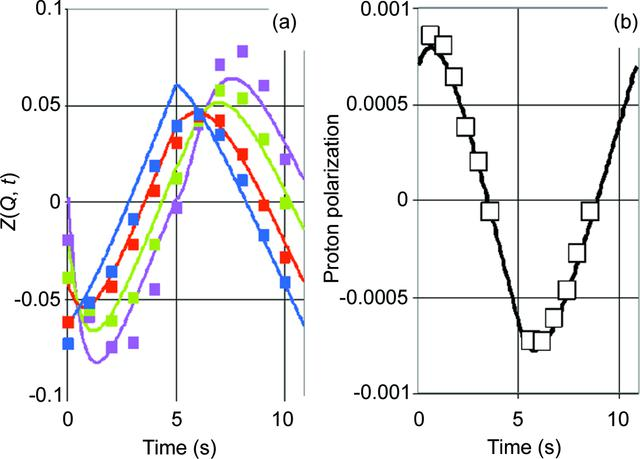disable zoom     view article Figure 18 Time-resolved neutron scattering Z(Q, t) at various Q, and the polarization of bulk protons versus time from EHBA–CrV (c = 1.53 × 1019 ml−1) in a mixture of glycerol/water. (a) Zexp(Q, t) is presented as two-dimensional Fourier series terminated at N1 = 1 and N2 = 1: pink 0.30, green 0.45, red 0.58 and blue 0.70, with the respective lines denoting Zcalc(Q, t). An average value of 620 a.u. has been subtracted from I(Q, t) to obtain Z(Q, t). (b) Open squares and the black line denote the bulk proton polarization from NMR and from Zcalc(Q, t), respectively.

IUCrJ
Volume 3| Part 5| September 2016| Pages 326-340
ISSN: 2052-2525